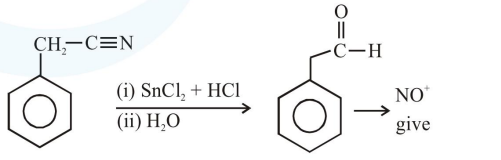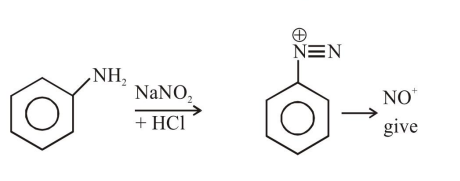# The Kjeldahl method of Nitrogen estimation fails

Question:

The Kjeldahl method of Nitrogen estimation fails for which of the following reaction products ?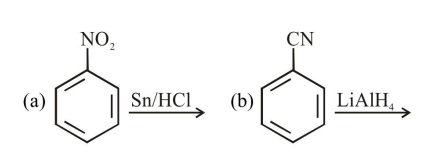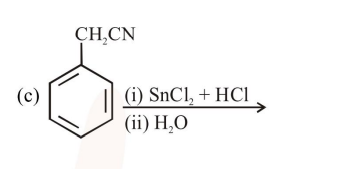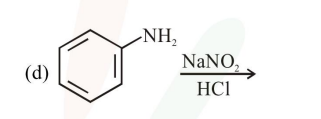1. a and d

2. c and $\mathrm{d}$

3. $\mathrm{a}, \mathrm{c}$ and $\mathrm{d}$

4. $b$ and $c$

Correct Option: 2,

Solution:

Kjeldahl method is used for $\mathrm{N}$ estimation But not given by 'Diazo' compounds